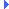FD.io VPP  v19.08-27-gf4dcae4 Vector Packet Processing
queue.h File ReferenceInclude dependency graph for queue.h:This graph shows which files directly or indirectly include this file:

Go to the source code of this file.

## Typedefs

typedef struct _svm_queue svm_queue_t

typedef svm_queue_t unix_shared_memory_queue_t

## Enumerations

enum  svm_q_conditional_wait_t { SVM_Q_WAIT = 0, SVM_Q_NOWAIT, SVM_Q_TIMEDWAIT }

## Functions

svm_queue_tsvm_queue_alloc_and_init (int nels, int elsize, int consumer_pid)
Allocate and initialize svm queue. More...

svm_queue_tsvm_queue_init (void *base, int nels, int elsize)

void svm_queue_free (svm_queue_t *q)

int svm_queue_add (svm_queue_t *q, u8 *elem, int nowait)

int svm_queue_add2 (svm_queue_t *q, u8 *elem, u8 *elem2, int nowait)

int svm_queue_sub (svm_queue_t *q, u8 *elem, svm_q_conditional_wait_t cond, u32 time)

int svm_queue_sub2 (svm_queue_t *q, u8 *elem)

void svm_queue_lock (svm_queue_t *q)

void svm_queue_unlock (svm_queue_t *q)

int svm_queue_is_full (svm_queue_t *q)

int svm_queue_add_nolock (svm_queue_t *q, u8 *elem)

int svm_queue_sub_raw (svm_queue_t *q, u8 *elem)

void svm_queue_wait (svm_queue_t *q)
Wait for queue event. More...

int svm_queue_timedwait (svm_queue_t *q, double timeout)
Timed wait for queue event. More...

void svm_queue_add_raw (svm_queue_t *q, u8 *elem)
Add element to queue with mutex held. More...

void svm_queue_set_producer_event_fd (svm_queue_t *q, int fd)
Set producer's event fd. More...

void svm_queue_set_consumer_event_fd (svm_queue_t *q, int fd)
Set consumer's event fd. More...

## Typedef Documentation

 typedef struct _svm_queue svm_queue_t

Definition at line 132 of file queue.h.

## Enumeration Type Documentation

Enumerator
SVM_Q_WAIT

blocking call - best used in combination with condvars, for eventfds we don't yield the cpu

SVM_Q_NOWAIT

non-blocking call - works with both condvar and eventfd signaling

SVM_Q_TIMEDWAIT

blocking call, returns on signal or time-out - best used in combination with condvars, with eventfds we don't yield the cpu

Definition at line 40 of file queue.h.

## Function Documentation

 int svm_queue_add ( svm_queue_t * q, u8 * elem, int nowait )

Definition at line 239 of file queue.c.Here is the call graph for this function:Here is the caller graph for this function:

 int svm_queue_add2 ( svm_queue_t * q, u8 * elem, u8 * elem2, int nowait )

Definition at line 289 of file queue.c.Here is the call graph for this function:Here is the caller graph for this function:

 int svm_queue_add_nolock ( svm_queue_t * q, u8 * elem )

Definition at line 192 of file queue.c.Here is the call graph for this function:Here is the caller graph for this function:

 void svm_queue_add_raw ( svm_queue_t * q, u8 * elem )

Add element to queue with mutex held.

Parameters
 q queue elem pointer element data to add

Definition at line 220 of file queue.c.Here is the call graph for this function:Here is the caller graph for this function:

 svm_queue_t* svm_queue_alloc_and_init ( int nels, int elsize, int consumer_pid )

Allocate and initialize svm queue.

Parameters
 nels number of elements on the queue elsize element size, presumably 4 and cacheline-size will be popular choices. pid consumer pid
Returns
a newly initialized svm queue

The idea is to call this function in the queue consumer, and e-mail the queue pointer to the producer(s).

The vpp process / main thread allocates one of these at startup; its main input queue. The vpp main input queue has a pointer to it in the shared memory segment header.

You probably want to be on an svm data heap before calling this function.

Definition at line 72 of file queue.c.Here is the call graph for this function:Here is the caller graph for this function:

 void svm_queue_free ( svm_queue_t * q )

Definition at line 89 of file queue.c.Here is the call graph for this function:Here is the caller graph for this function:

 svm_queue_t* svm_queue_init ( void * base, int nels, int elsize )

Definition at line 33 of file queue.c.Here is the call graph for this function:Here is the caller graph for this function:

 int svm_queue_is_full ( svm_queue_t * q )

Definition at line 109 of file queue.c.

 void svm_queue_lock ( svm_queue_t * q )

Definition at line 97 of file queue.c.Here is the caller graph for this function:

 void svm_queue_set_consumer_event_fd ( svm_queue_t * q, int fd )

Set consumer's event fd.

When the consumer must generate an event it writes 1 to the provided fd. Although in practice the two fds point to the same underlying file description, because the producer and consumer are different processes the descriptors will be different. It's the caller's responsibility to ensure the file descriptors are properly exchanged between the two peers.

Definition at line 469 of file queue.c.

 void svm_queue_set_producer_event_fd ( svm_queue_t * q, int fd )

Set producer's event fd.

When the producer must generate an event it writes 1 to the provided fd. Once this is set, condvars are not used anymore for signaling.

Definition at line 463 of file queue.c.

 int svm_queue_sub ( svm_queue_t * q, u8 * elem, svm_q_conditional_wait_t cond, u32 time )

Definition at line 348 of file queue.c.Here is the call graph for this function:Here is the caller graph for this function:

 int svm_queue_sub2 ( svm_queue_t * q, u8 * elem )

Definition at line 413 of file queue.c.Here is the call graph for this function:Here is the caller graph for this function:

 int svm_queue_sub_raw ( svm_queue_t * q, u8 * elem )

Definition at line 443 of file queue.c.Here is the caller graph for this function:

 int svm_queue_timedwait ( svm_queue_t * q, double timeout )

Timed wait for queue event.

Must be called with mutex held.

Parameters
 q svm queue timeout time in seconds
Returns
0 on success, ETIMEDOUT on timeout or an error

Definition at line 183 of file queue.c.Here is the call graph for this function:Here is the caller graph for this function:

 void svm_queue_unlock ( svm_queue_t * q )

Definition at line 103 of file queue.c.Here is the caller graph for this function:

 void svm_queue_wait ( svm_queue_t * q )

Wait for queue event.

Must be called with mutex held.

Definition at line 151 of file queue.c.Here is the call graph for this function:Here is the caller graph for this function: